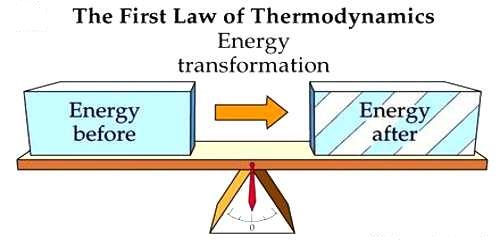Physics

# Significance of the First Law of ThermodynamicsSignificance of the First Law of Thermodynamics

Thermodynamics is the branch of physics that deals with the relationships between heat and other forms of energy. The first law of thermodynamics is a special form of the principle of conservation of energy. The law of conservation of energy states that the total energy of an isolated system is constant; energy can be transformed from one form to another, but cannot be created or destroyed.

Energy cannot be formed nor ruined. The universe can never have more energy than it started with after the big bang. It also brings into matter the big bang. Before it out the universe had no energy, now we have all of it. How did that huge amount of energy break into our universe? It also brings into the play the thought that energy cannot be destroyed. However, it can be used, (by the law of entropy) to a state that it cannot be reutilized into a helpful form. In quintessence, the amount of energy that humans can work with will ultimately shrink to nothing, even though the amount of energy is similar as it was in the beginning.

The first law of thermodynamics has the following significances:

• It establishes the relation between heat and work. The total energy of an isolated system must remain constant.
• According to this law, a fixed amount of heat is needed to get a fixed amount of work or to get a fixed amount of heat a fixed amount of work is needed.
• It is impossible to get work or energy without expending something.
• Work and heat are equivalent to each other.
• It is nothing but the principle of conservation of energy. In any system, the sum of work done and change in internal energy is always equal to supplied heat.
• If the system gained or loss energy then exactly the equivalent amount of energy of surrounding will have a loss or gain.
• No engine has been built which is able to perform work without expending fuel or energy, i.e., it is not possible to invent the perpetual motion machine which can perform work without expending energy.
• You cannot have a device which gives output as work without any heat input. This device is known as a perpetual motion machine of the 1st kind which is not probable.
• The first significance is related to the law of conservation of energy, heat, and work both are different forms of the same entity known as energy which is conserved.
• Whenever a definite quantity of one kind of energy disappears, an accurately correspondent amount of some other kind must appear.
• The energy of an isolated system is constant. Example: Universe